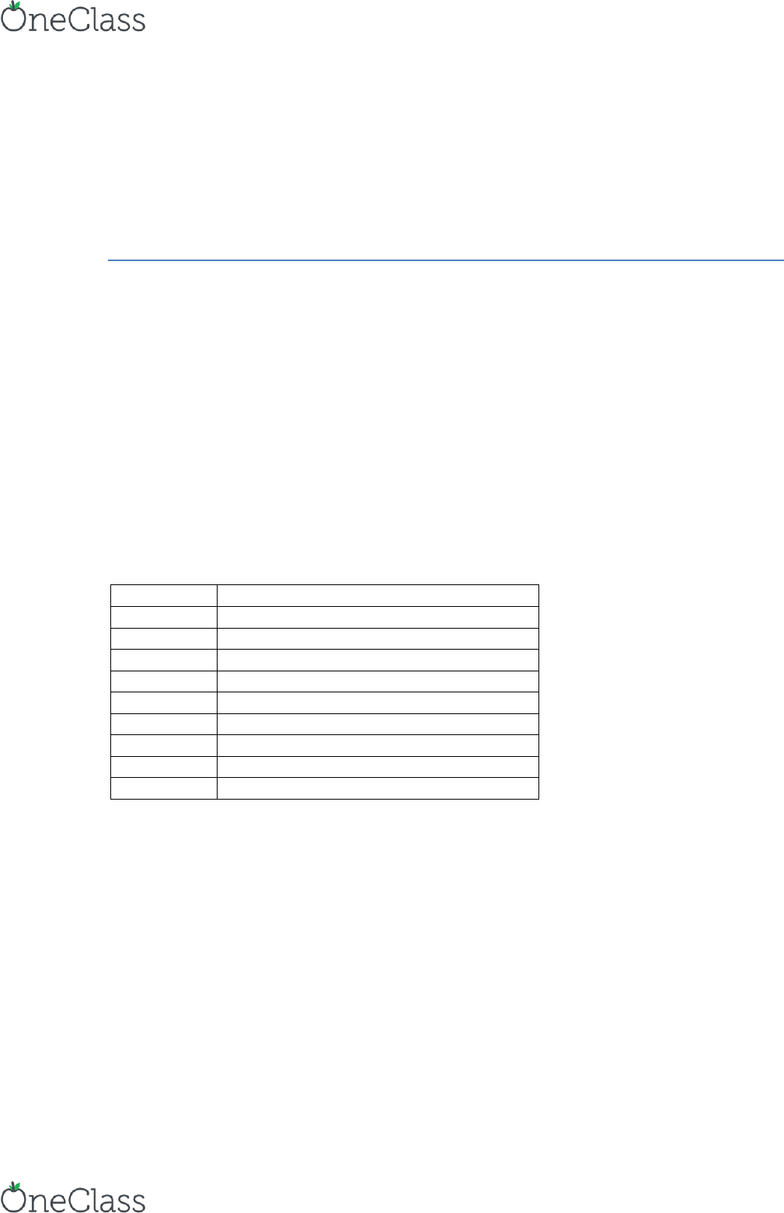# ENGRMAE 106 Lecture Notes - Lecture 3: Low-Pass Filter, High-Pass Filter, Rc Circuit

18 views11 pages
Page:
of 11MAE106 Laboratory Exercises
Lab # 2 - Introduction to data
acquisition systems and filters
University of California, Irvine
Department of Mechanical and Aerospace Engineering
Goals
To learn how to read an analog signal into a microcontroller and process it, then send
out a signal.
To learn about analog and digital filters and how they can be applied to robotics and
To observe the dynamics of a first-order system.
Parts & equipment
Qty
Part/Equipment
1
2
10KOhm Potentiometers
Various
Wire
1
Function generator (Trainer kit)
1
Oscilloscope
1
Multimeter
1
Seedduino board
1
Capacitor
find more resources at oneclass.com
find more resources at oneclass.com
Introduction (Pre-Lab)
When dealing with electromechanical systems we often need to read signals from sensors
that can convert physical phenomena into electrical signals (i.e. potentiometers,
acceloremeters, thermometers, etc.), process them, and take actions based on these
readings. A key part of this process involves being able to read these analog signals and
transform them into digital ones so that a computer can manipulate them. To do this, we
will use an inexpensive data acquisition system (DAQ): the Seeeduino microcontroller.
In a common configuration for DAQ systems (Figure 1) - and one we will be using
throughout this class - we first want to read from one or multiple sensors. We will do so
by using the DAQ system (i.e. seeeduino) to convert the analog (i.e. continuous) signal
into a digital (i.e. 0's and 1's) signal; this is known as analog-to-digital (A/D) conversion.
Once the signal is read into the microcontroller we can now process it and finally create
new signals to control outputs such as lights or motors; this is known as digital-to-analog
(D/A) conversion.
Part I: Analog filters and Pulse-width modulation
In many cases when dealing with real-world
systems the input and output signals that
we use will require some type of pre- and/or
post-processing. A key tool in this
processing is filtering.
Signal processing filters are an important
part of engineering design. They are often
found in stereos (cross-overs, graphic
equalizers, etc.), control systems (for cars
and planes, to clean up sensor readings from strain gauges, tachometers, potentiometers,
etc.), and many other applications. In control systems, filters can help remove unwanted
high-frequency noise that may adversely affect the controller. Understanding how systems
respond to different input frequencies requires understanding how filters work. So, in
summary, as a mechanical engineer designer, it is likely that you will want to use filters
In this lab, we will study the RC circuit, which can be used as a low-pass or high-pass
filter. Low-pass filters attenuate high frequency signals (i.e. reduce them in amplitude),
but leave low frequency signals relatively unchanged. Low pass filters are often useful for
filtering out high-frequency noise (due to electromagnetic interference from the lights or
Figure 1. Common configuration for a DAQ system
Figure 2: Low-Pass Filter
find more resources at oneclass.com
find more resources at oneclass.com
radio signals, for example.) In contrast, high pass filters attenuate low frequency signals.
They are used in many applications as well, such as filtering low frequency “drift” from
electrodes measuring muscle or brain activity.
First-Order Linear Systems: This lab also provides a chance for you to measure how a
first-order linear system behaves in the time and frequency domains. The RC circuit is a
first-order system (i.e. it is described by a first-order differential equation). The
mathematics behind a first-order system are extremely common in engineering, so
measuring an actual first-order system will give you experience and intuition about how
any first-order system behaves, whether it be electrical, mechanical, chemical, or
biological.
RC Low-pass filter description
The circuit shown in Figure 2 is a low-pass filter. Thus, it tends to get rid of any high
frequencies on the input Vin, just allowing low frequencies through to the output Vout. To
get some physical intuition why, consider the response of the circuit a square wave voltage
input. A square wave is a signal that changes very rapidly for short periods of time (when
the voltage jumps up, or when it jumps down). Based on Fourier analysis, in which we
view a signal as a sum of sinusoids at different frequencies, we know that these rapid
changes are enabled by very high frequency sinusoids contained in the square wave.
The RC circuit filters out these high frequency sinusoids, and therefore the rapid changes.
The capacitor (C) acts as a charge bucket, which is alternately charged (filled) and
discharged (emptied) by the square wave input Vin. Essentially, the capacitor is charged
by Vin until the voltage across the capacitor matches that of Vin. If Vin is then switched
off to a lower voltage value, C begins to discharge through R so that Vout heads toward
that low value again. It takes time, however, for the capacitor to charge and discharge
through the resistor. That is, the capacitor has dynamics that slow down Vout and prevent
it from exactly following Vin.
RC Low-pass filter Arithmetic
The differential equation that relates Vout to a step change in Vin can be derived from
KCL and KVL for the RC circuit and is given by:
    (1)
where =RC is the time constant of the system. Thus, because of the first order
dynamics, the net effect of the low-pass filter is to get rid of the rapid transitions in voltage
in the square wave.
find more resources at oneclass.com
find more resources at oneclass.com